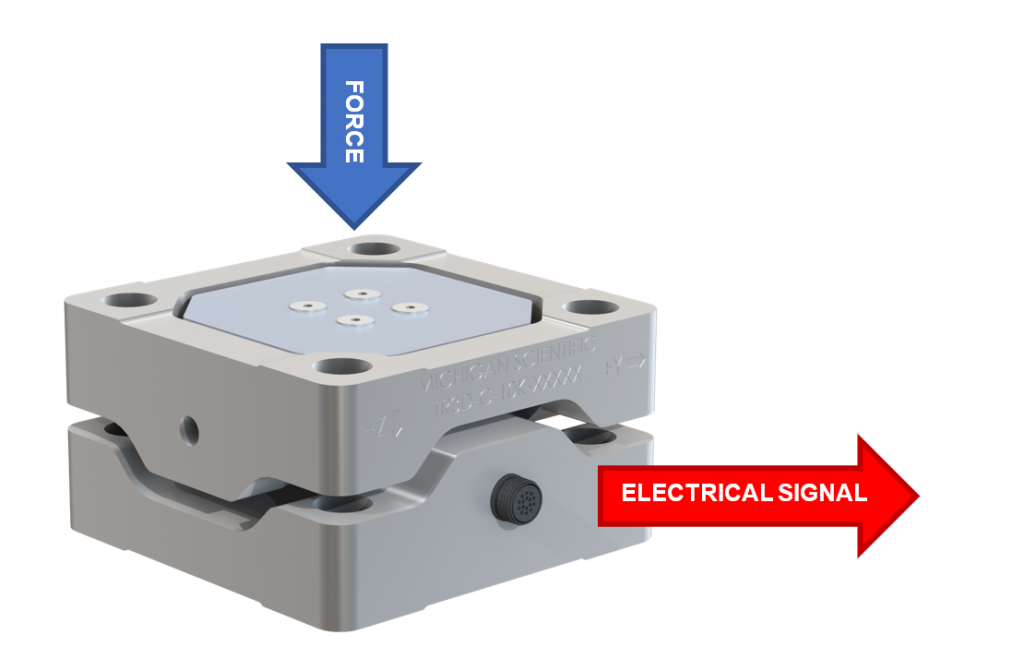Understanding force measurement and the devices used for it, isn’t particularly difficult. All you need is an understanding of the basics of load cells, and a handy guide to give you that knowledge. Here at Michigan Scientific, we’ve got you covered.

Let’s explore more about load cells—what they are, how they work, and when you might use one.

Load cells can be used to detect when an object exerts a force. You can also use them to track the changes in the force applied over time. In some applications, load cells are used to detect the presence of a force by measuring the strain on a surface due to the input. Strain is a deformation response on a surface due to an applied load. When a force is applied, the load cell converts this energy to an electrical signal.Load cells are physical elements, also known as transducers. Transducers can convert one form of energy into another form of energy; meaning if you apply force into a load cell, it can be transformed into an electrical signal.

This signal will have a magnitude that is directly proportional to the force being applied, making it easier to measure.

## What are the Types of Load Cells?

There are various types of load cells. The three most common ones are strain gauge, hydraulic, and pneumatic load cells. This means that there are three easy ways for a load cell to translate an applied force into a measurable reading. Michigan Scientific specializes in both stock strain gauge load cells, and custom strain gauge load cells.

And while there are other types of load cells, they are less commonly applied.

### Strain Gauge Load Cells: Mechanical Element

Strain gauge load cells are mechanical in nature. This mechanical element makes use of a strain gauge, or multiple strain gauges, in order to sense a deformation caused by the force applied.

Strain gauges often vary in size, production materials, and even mechanical setup in order to accommodate different loads. They can also have varying sensitivities based on their intended purpose. Despite having different designs, all strain gauges work on the same concept, turning deflection into a change in resistance.

Common strain gauges are made up of a very fine wire or foil in a grid pattern, wherein a linear change in electrical resistance can be caused by detecting strain due to force, weight, or pressure in a specific direction. There is often a base resistance of 120Ω, 350Ω, or 1,000Ω.

As noted above, every strain gauge can have different levels of sensitivity. This is expressed as the “gauge factor,” or GF, and is defined as the ratio of fractional change in electrical resistance to the fractional deformation in the direction the force is applied (or strain).

Gauge Factor = GF = (∆R/R)/(∆L/L) = (∆R/R)/ε, where ε = strain

### Hydraulic Load Cells: Piston and Cylinder Setup

Hydraulic load cells, on the other hand, make use of conventional cylinder and piston arrangements in order to show the change in pressure. The movement of the piston can help determine the weight of the object by measuring the change in pressure on a connected Bourdon tube.

### Pneumatic Load Cells: Using Air Pressure

Lastly, pneumatic load cells make use of air pressure to get the same results. Air pressure is applied to one end of a diaphragm, which then escapes through the nozzle found at the bottom of the load cell.

Both hydraulic and pneumatic load cells work well in a lab environment with static loads. Strain gauge load cells are very useful for dynamic loads and in-field testing.